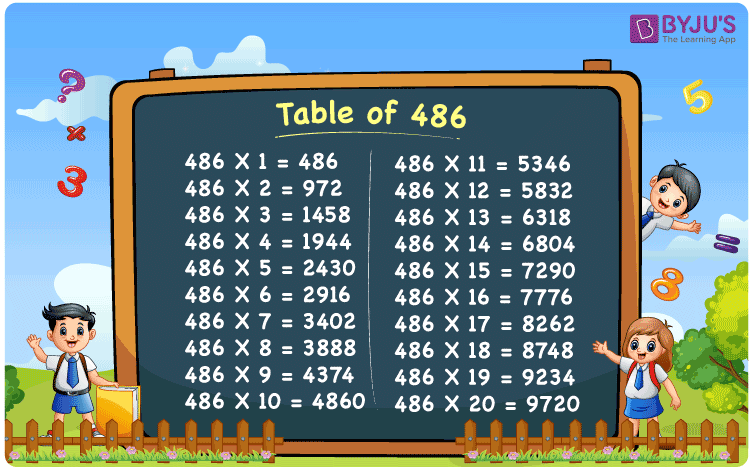Checkout JEE MAINS 2022 Question Paper Analysis : Checkout JEE MAINS 2022 Question Paper Analysis :

# Table of 486

When the number 486 is multiplied by a natural number, the Table of 486 displays repeated addition. For example, multiplying 486 by 3 becomes 486 + 486 + 486 = 1458. As a result, 486 x 3 equals 486. Similarly, we can write the 486 times table up to 20 in this article. The multiplication table can be used to quickly solve arithmetic problems. The table of 486 can also be downloaded from the link provided here.

## Table of 486 Chart## What is the 486 Times Table?

As previously mentioned, the table of 486 shows the addition of 486 several times when multiplied by another number. You will learn how to frame the 486 times table in the sections below.

 486×1 = 486 486 486×2 = 972 486 + 486 = 972 486×3 = 1458 486 + 486 + 486 = 1458 486×4 = 1944 486 + 486 + 486 + 486 = 1944 486×5 = 2430 486 + 486 + 486 + 486 + 486 = 2430 486×6 = 2916 486 + 486 + 486 + 486 + 486 + 486 = 2916 486×7 = 3402 486 + 486 + 486 + 486 + 486 + 486 + 486 = 3402 486×8 = 3888 486 + 486 + 486 + 486 + 486 + 486 + 486 + 486 = 3888 486×9 = 4374 486 + 486 + 486 + 486 + 486 + 486 + 486 + 486 + 486 = 4374 486×10 = 4860 486 + 486 + 486 + 486 + 486 + 486 + 486 + 486 + 486 + 486 = 4860

## Multiplication Table of 486

The table of 486 up to 20 times can be found below. We can use this table to answer multiplication problems.

 486 × 1 = 486 486 × 2 = 972 486 × 3 = 1458 486 × 4 = 1944 486 × 5 = 2430 486 × 6 = 2916 486 × 7 = 3402 486 × 8 = 3888 486 × 9 = 4374 486 × 10 = 4860 486 × 11 = 5346 486 × 12 = 5832 486 × 13 = 6318 486 × 14 = 6804 486 × 15 = 7290 486 × 16 = 7776 486 × 17 = 8262 486 × 18 = 8748 486 × 19 = 9234 486 × 20 = 9720

## Solved Example on the Table of 486

Q. Determine the value of 486 x 5 + 20.

Solution: Given,

486 x 5 + 20

= 2430 + 20

= 2450

## Frequently Asked Questions on the Table of 486

### What is 486 times 8?

The value of 486 times 8 is 486 × 8 = 3888.

### What is the value of 486 multiplied by 11?

5346 is the result of multiplying 486 by 11. In the table of 486, 486 x 11 = 5346

### Why is the table of 486 used?

The table of 486 is a multiplication table that shows how many times a number has been multiplied by 486. It can be used in a variety of arithmetic calculations.